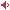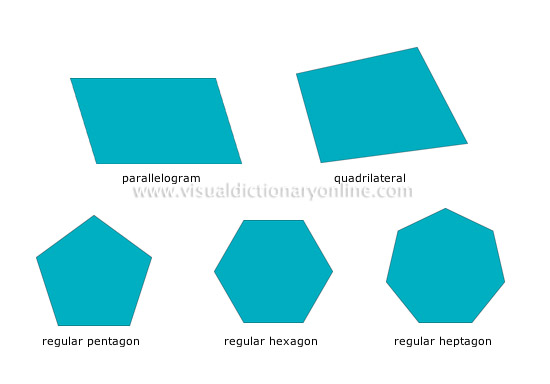# Visual Dictionary Online

Theme

#### polygonsGeometric plane figures with several sides and a number of equal angles.#### regular heptagonPolygon with seven (hepta = seven) sides and equal angles.

#### regular pentagonPolygon with five (penta = five) sides and equal angles.

#### quadrilateralAny plane figure with four sides and four angles.

#### regular hexagonPolygon with six (hexa = six) sides and equal angles.

#### parallelogramTrapezoid whose opposite sides are parallel and of equal length; the sides do not meet at right angles.